﻿ The Standard Representation of the Symmetric Group <i>S</i><SUB><i>n</i></SUB><i> </i>over the Ring of Integers

### The Standard Representation of the Symmetric Group Snover the Ring ...

Kunle Adegoke, Olawanle Layeni, Rauf Giwa, Gbenga Olunloyo

Turkish Journal of Analysis and Number Theory

## The Standard Representation of the Symmetric Group Snover the Ring of Integers

Kunle Adegoke1,, Olawanle Layeni2, Rauf Giwa1, Gbenga Olunloyo1

1Department of Physics and Engineering Physics, Obafemi Awolowo University, Ile-Ife, Nigeria

2Department of Mathematics, Obafemi Awolowo University, Ile-Ife, Nigeria

### Abstract

In this paper we give a Casimir Invariant for the Symmetric group Sn. Furthermore we obtain and present, for the first time in the literature, explicit formulas for the matrices of the standard representation in terms of the matrices of the permutation representation.

• Kunle Adegoke, Olawanle Layeni, Rauf Giwa, Gbenga Olunloyo. The Standard Representation of the Symmetric Group Sn over the Ring of Integers. Turkish Journal of Analysis and Number Theory. Vol. 3, No. 5, 2015, pp 126-127. http://pubs.sciepub.com/tjant/3/5/3
• Adegoke, Kunle, et al. "The Standard Representation of the Symmetric Group Sn over the Ring of Integers." Turkish Journal of Analysis and Number Theory 3.5 (2015): 126-127.
• Adegoke, K. , Layeni, O. , Giwa, R. , & Olunloyo, G. (2015). The Standard Representation of the Symmetric Group Sn over the Ring of Integers. Turkish Journal of Analysis and Number Theory, 3(5), 126-127.
• Adegoke, Kunle, Olawanle Layeni, Rauf Giwa, and Gbenga Olunloyo. "The Standard Representation of the Symmetric Group Sn over the Ring of Integers." Turkish Journal of Analysis and Number Theory 3, no. 5 (2015): 126-127.

 Import into BibTeX Import into EndNote Import into RefMan Import into RefWorks

### 1. Introduction

The symmetric group Sn, whose elements consist of the set of all permutations on n symbols is of central importance to mathematics and physics . Cayley’s theorem states that every group is isomorphic to a subgroup of the symmetric group on that group. In physics the classification of atomic and nuclear states depends essentially on the properties of Sn . The representation theory of the symmetric group is a well studied subject [1, 2, 3]. The partitions of n or equivalently Young diagrams of size n are the natural ways in which to parametrize the irreducible representations of Sn . This paper is concerned, not with the general irreducible representations of Sn, but, more specifically, with the so-called standard representation of the symmetric group, formally obtained from the n-1 dimensional subspace of vectors whose sum of coordinates is zero in the basis set of a permutation representation. The path taken in this work shall however be non-group theoretic. For example, we will not be concerned with Young diagrams.

The standard representation of Sn is important for the following reason: For n7, the permutation representation, the trivial (identical) representation, the sign representation, the standard representation and another n-1 dimensional irreducible representation found by tensoring with the sign representation are the only lowest-dimensional irreducible representations of Sn . All other irreducible representations have dimension at least n. While it is a fact that all irreducible representations of Sn can be found, using Frobenius formula (, pp 189), for example, there are no known explicit formulas for the standard representation. The main result of this paper is the derivation of such formulas, which now make it possible to write down the standard representation matrices directly from those of the permutation representation.

### 2. The Permutation Representation

Denote the n! elements of Sn by, such that, in usual notation,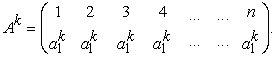(1)

and, allbeing distinct. For simplicity, and since no ambiguity can result, we will use the same symbolfor the representation matrices. Then, in the permutation representation, the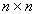matrices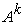are given, through their elements, by: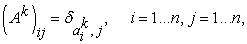(2)

which is clearly a unitary representation, since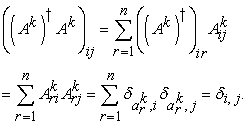2.1. A Casimir Invariant for Sn in the Permutation Representation

Theorem 1. Thematrix C, with elements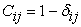is a Casimir Invariant of Sn in the permutation representation.

Proof We require to prove that C commutes with every.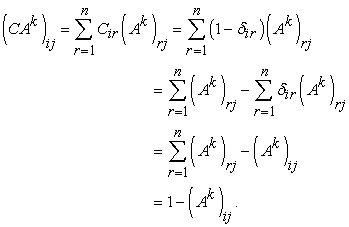(3)

A similar calculation gives,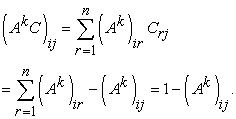(4)

We see, therefore, that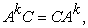so that C is a Casimir Invariant of the symmetric group.

### 3. The Standard Representation

Since the Casimir invariant C, obtained in the previous section, is not proportional to the identity, Schur’s lemma tells us that the permutation representation is not irreducible, a well-known fact. It therefore remains to find the matrix P which diagonalizes C. First we prove a lemma.

Lemma 1 The nonsingularmatrix P with elementshas the inverse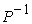where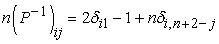Proof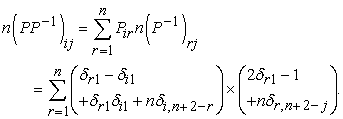(5)

It is straightforward to write out the terms and evaluate the summation termwise. One merely needs to note that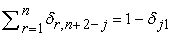andOne then finds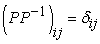, which establishes the claim.

3.1. Diagonal form of the Casimir Invariant

Theorem 2. The matrix P given in Lemma 1, diagonalizes the Casimir Invariant, C.

Proof. We wish to computeNow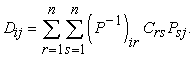Substituting the matrix elements, expanding and evaluating the sums, we find after some algebra, that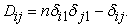(6)

Thus we see that D is a diagonal matrix, as claimed, with the entry ‘n1’ in row 1, column 1 and the remaining diagonal elements being 1.

The matrix P, above, which diagonalizes C will block-diagonalize the matrices.

3.2. Similarity Transformation of Ak: The Standard Representation

Using the matrix elements of P, P1 and Ak, it is not difficult to obtain the interesting result: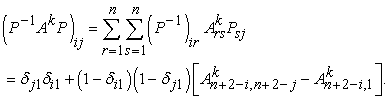(7)

We see from (7) that each matrixis block diagonal, being the direct sum of amatrix with entry 1 and an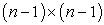matrix Bk, with elements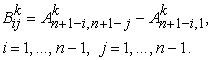(8)

The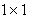matrices correspond to the identical (trivial) representation in which every element of Sn is sent to the one-dimensional identity matrix, while the Bk matrices correspond to the irreducible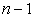dimensional standard representation.

### 4. Conclusion

In this paper we have shown that the operator C with matrix elementsis a Casimir Invariant for the symmetric group Sn. We also showed that if,are the representation matrices for the elements of Sn in the permutation representation, then the matrices Bk for the standard representation of Sn are given by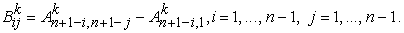### References

  M. Hamermesh. Group Theory and Its Application to Physical Problems. Dover Publications, 1989.In article  W. Burnside. Theory of groups of finite order. Dover Publications, 1955.In article PubMed  W. Fulton and J. Harris. Representation theory. A first course,. Springer-Verlag, 1991.In article  Wikipedia. Representation theory of the symmetric group http://en.wikipedia.org/wiki/Representation_theory_of_the_symmetric_group. 2015.In article